Punch cards were taken over by software a long time ago. There are all kinds of applications, proprietary software, and templates that help businesses track their employees and bill their clients. However, most professionals swear by the most reliable office tool available (hint: Excel) and build such tools in-house. Billing clients or tracking employee hours can differ greatly for various industries and applications that help this process are rarely one-size-fits-all.

In this article we will be focusing on creating your own time card using Microsoft Excel. Although this should give you a pretty good idea about the points you should consider when building such a tool, feel free to optimize it for your business case.

One of your first considerations should be the timeframe. Typically, companies collect data and pay their employees weekly, biweekly, or monthly. In this tutorial, we’re going to create a weekly time card. Another question would be whether you want to include a lunch break, as this will determine the general layout and a few formulas in this tool.

### Layout

First off, let’s start with creating the labels, and resizing the columns and rows to match text size. We’re going to need a title section where we display the time-card specific information including name of the employee, department, and week of the year. Then, we need a table to input dates, days of the week, and in/out times. You can add as much detail as you want. If you'd like to use the spreadsheet we've created as a guideline, please feel free to download it here.We are going to be using two sets of Time In and Time Outs to allow for a lunch break in between. An overview of the time offs and how the times add up will come in handy when reading the table.We can pull the Day of Week from the Date column. To do this, we can make column B (B6:B12) equal to A (A6:A12) and then change the formatting. Simply type in =A6 into cell B6 and press Enter. Then, do the same for rows until 12. Select the cells in the B column (B6:B12) and right click. From the menu, select Custom for Category and dddd for Type.Press Ok. All dates in column B will now only display day of the week.Next, we’re going to create lists to build time selection lists. We can do this by adding an Hours and a Minutes column for each Time In/Out. This way, we guarantee that users enter times in the correct format. Let’s add a new sheet (Options sheet in our example) and create two columns of numbers; Hours (0 to 23) and Minutes (0 to 59).We’re going to use Data Validation to create combo box type of selectors for time fields. First, select the first Hour cell (C6), then go to the Excel ribbon Data. Here, select Data Validation and select List from the Allow menu. Make sure the Source is targeting our Hour list by pointing this field there (=Options!\$D\$2:\$D\$25).Then, do the same thing for the time selections. Go to the second sheet where we added the Hour and Minutes columns and select them both. Right click, and go to Format Cells menu. Select Custom and format as “00”.Now, we need to copy this cell group into all relevant time fields. Copy C6:D6 to the C6:J12 range. The table should now look like this,### Calculations

The beauty and one of the main reasons why businesses still use Excel for time card applications is its flexibility. We can add any type of logic through formulas. For instance, let’s use the column Regular to check whether the regular work hours were satisfied on a given day. Here, we need to enter a formula to add up the hours coming from the previous columns. Assuming a regular work day is 8 hours, we can insert an IF statement to display the total hours, or make it obvious that the requirement wasn’t met.

Go to L6 and add this formula,

=IF(((E6+F6/60)-(C6+D6/60))+((I6+J6/60)-(G6+H6/60))>8,8,((E6+F6/60)-(C6+D6/60))+((I6+J6/60)-(G6+H6/60)))

Then, dragging the lower right corner of this cell, apply the same logic to consecutive rows in this column. We should have this formula applied to the L6:L12 range.

Let’s break this down. The first part of the formula (((E6+F6/60)-(C6+D6/60))+((I6+J6/60)-(G6+H6/60))), sums everything up, finds the difference between Time In and Time Outs, and subtracts them from the total. The “/60” divisions are for converting the second columns (minutes) to hours. The second part of the formula checks to see if the difference is greater than “8”. If hours add up to 8, it prints “8”, if it’s less, the formula gives the exact sum.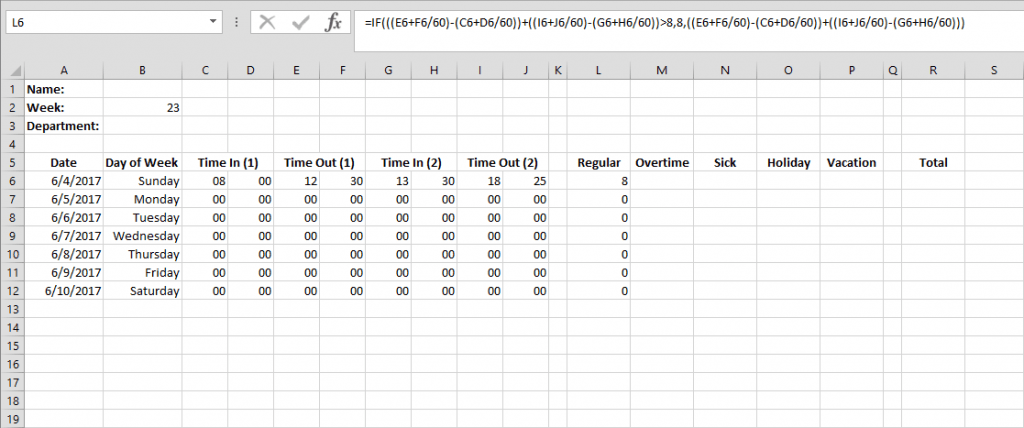Calculating Overtime can be done using a similar formula. Enter this formula to cell M6,

=IF(((E6+F6/60)-(C6+D6/60))+((I6+J6/60)-(G6+H6/60))>8,((E6+F6/60)-(C6+D6/60))+((I6+J6/60)-(G6+H6/60))-8,0)

Apply the same logic to all consecutive cells in this column. The first part of the formula, again, adds up the Time In/Outs, and subtracts the breaks. The second part prints the overtime hours if the difference is greater than 8. If not, it gives “0”.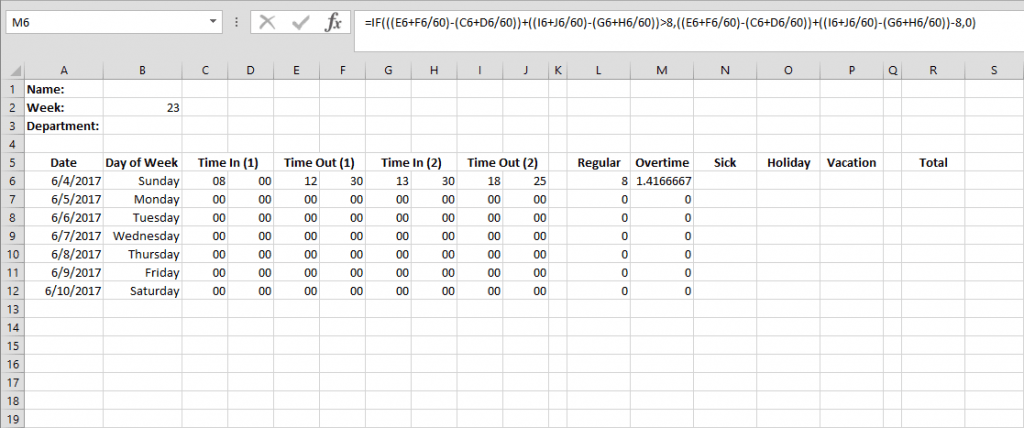Adding up the total hours, including all time offs will give us the daily totals. We can use a simple SUM formula to calculate this. Next, let’s add,

=SUM(L6:P6)

To R6 and apply the same logic to the following rows until R12.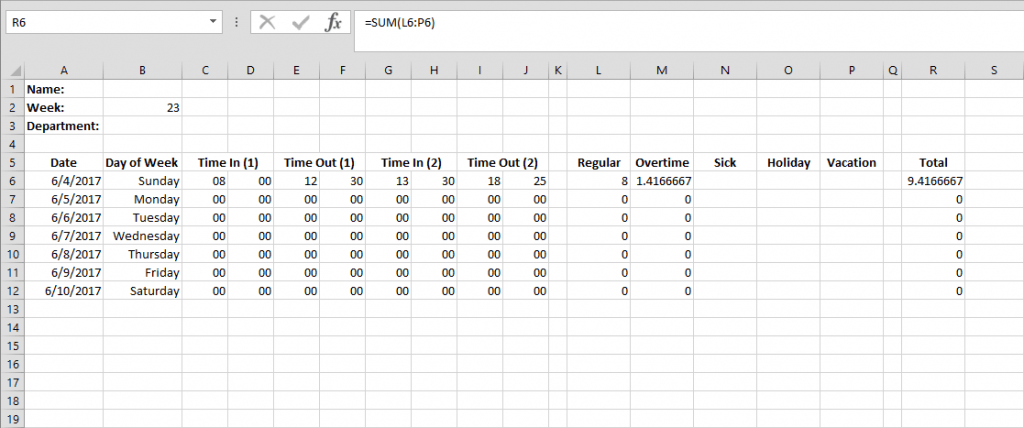Finding the weekly totals can be done in the same fashion. Let’s again use a SUM formula,

=SUM(L6:L12)

And show the total in L13 and the following columns, until R13.We’re done with the calculations! All that is left to do is adding default values and complement the looks with some formatting. Enter zeros for all fields under Sick, Holiday, and Vacation columns.Next, we need to match the formatting and get rid of floating decimals. Select the entire range of numbers from column L, to column R. Right click the selection and go to Format Cells. Select Number for format type and make sure decimals are rounded to two figures.Almost there! Let’s make everything look pretty and easier to read by adding some highlight colors and borders. This part is entirely up to you. We choose to use this design,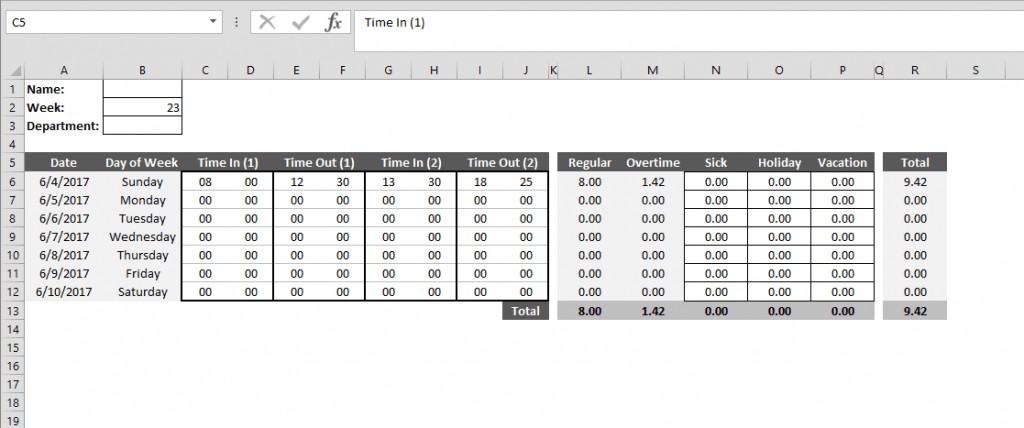We are pretty much done at this point and the rest of this guide focuses on additional features. Let’s continue with adding Hourly Wage and Total Pay calculations. Create a new inputs section in row 13 and labels section in row 16 and cover the columns L through P. L13 through P13 will be Rate/Hr inputs for hourly pays, and L16 through P16 will give the Total Pay for the week. Simply enter

=L13*L15

To cell L16 and apply the same logic through P16.Finally, add a Grand Total cell to add up all pays. Enter

=SUM(L16:P16)

To cell L18.

Data cleanliness is one of the primary concerns in Excel based tools. Without additional measures, there’s nothing preventing users from entering “-1” for the week of the year. Input validation can ensure that only a certain range of inputs are allowed into specified fields. Let’s start with applying this to Week of the year. We first start with calculating the maximum number of weeks in the current year (leap years have 53 weeks).

=WEEKNUM(DATE(YEAR(TODAY()),12,31),21)

Enter this formula into an empty cell in our Options sheet where we’re keeping the time lists. WEEKNUM is a handy function that returns the week of the year and it can take 2 arguments, the first one date, and the second is optional value. We recommend using “21” as return type to follow ISO standard for printing dates.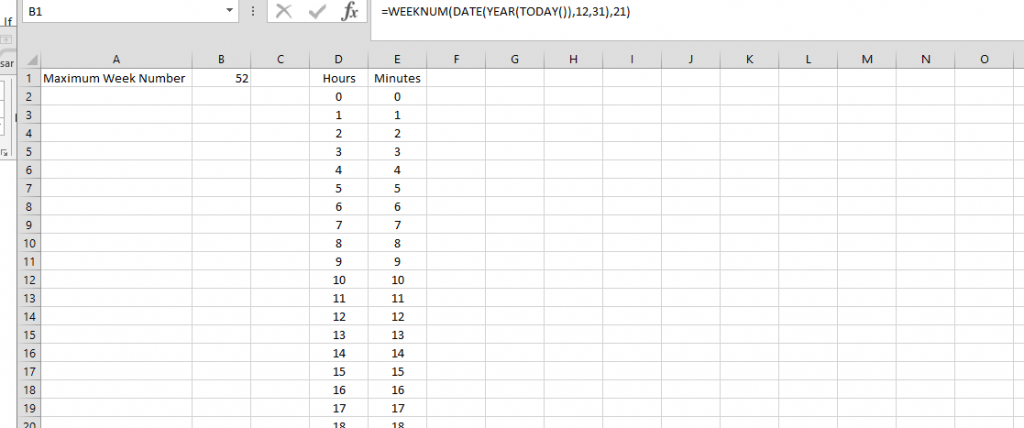Select the input Week (B2) and go to Data ribbon in Excel menu. Select Data Validation and apply the following settings:

• Allow: Whole Number
• Data: Between
• Minimum: 1 (no more “-1” weeks)
• Maximum: Options!B1(or wherever you placed the weeks formula)

Furthermore, we can apply the same logic (minus the maximum weeks) to Sick, Holiday, Vacation, and Wage inputs.

Wouldn’t it be amazing if the spreadsheet highlighted days that are below the regular hours? Luckily, Excel has a built-in feature called conditional formatting, through which we can do exactly that. Let’s mark days that fell below the 8 hour limit.

Begin with selecting the rows 6 to 12.

Then go to Home in the top Excel menu and press Conditional Formatting. Select New Rule and choose the option Use a formula to determine which cells to format. Enter

= AND(\$L6>0,\$L6<8)

Into the formula box. This function will check cells in column L to see if they are greater than “0”. Weekends, where these values are “0” will be ignored, but work days where less than 8 hours was entered will be selected. Now, select a format that you want the outliers to take. We choose the font color red.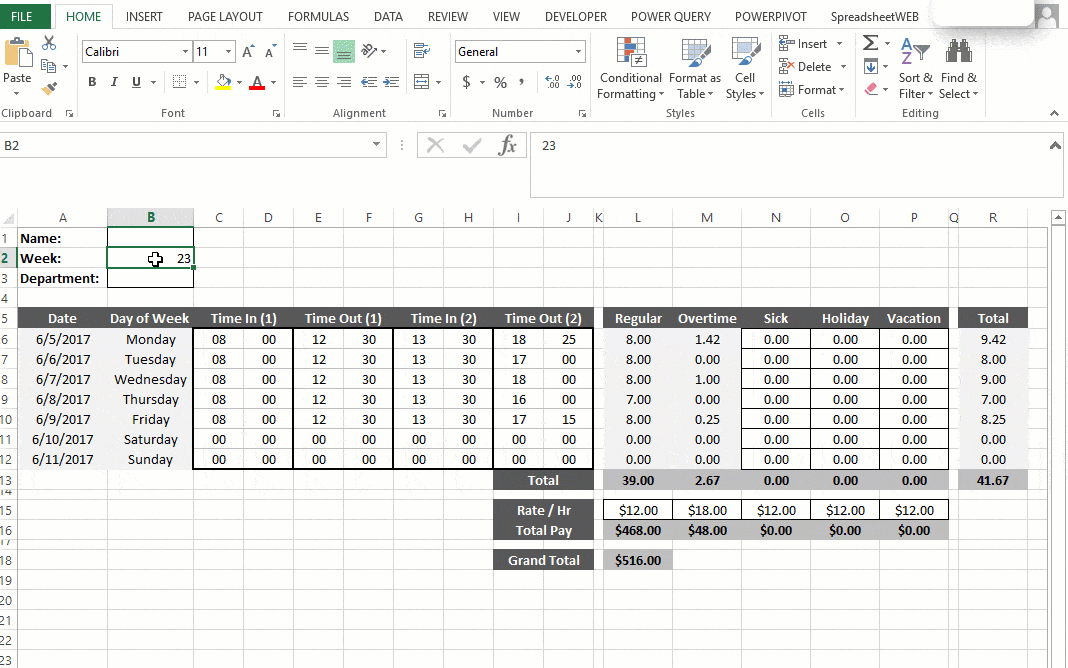Our time card is ready! We created a data collection application with a neat layout and automated the process of paying employees. Users can put in their times into this spreadsheet and find out about their pays. Likewise, management can collect this data in a clean form and will have perfect insight of business performance.